# Pythagorea Level 16.1 16.2 16.3 16.4 16.5 16.6 16.7 16.8 16.9 16.10 16.11 16.12 16.13 16.14 16.15 16.16 Solution/AnswersPythagorea is android/iOS app developed by Horis International Limited. Solutions hints and answers to pythagorea are available in this post scroll down to find solutions to all the levels.

This game is mostly focused on geometric puzzles and construction. the workspace is divided into grids to draw lines. You should know all the basic Math operations. All lines and shapes are drawn on a grid whose cells are squares. Most of the game levels can be answered using natural intuition and bye some basic laws of geometry.

You have to connect points on the grid using straight lines to construct an element, you can even use intersection points to draw. some levels are very easy some are of medium difficulty and some are very hard to solve, that’s why I am providing solutions to all the problems.

If you have forgotten basic course you did in your elementary education, this game is for you to revise all the concept using a single game or if you don’t know any of the geometry this game features “i” button from where you can learn about all the shapes and geometry and then play this game to enhance your geometry skill.And do not miss your chance to familiarize children with mathematics. Pythagorea is an excellent way to make friends with geometry and benefit from spending time together.

If you are here for levels other than ‘Angle Bisectors’ Go to directory of all other levels at : http://www.puzzlegamemaster.com/pythagorea-all-answers-solutions/

• Pythagorea Level 16.1: Construct an angle bisector of the given angle.• Pythagorea Level 16.2: Construct an angle bisector of the given angle.• Pythagorea Level 16.3: Construct the incenter of the triangle.• Pythagorea Level 16.4: Construct an angle bisector of the given angle.• Pythagorea Level 16.5: Construct the incenter of the triangle.• Pythagorea Level 16.6: Construct an angle bisector of the given angle.• Pythagorea Level 16.7: Construct an angle bisector of the given angle.• Pythagorea Level 16.8: Construct a bisector of the angle Whose vertex lies outside the field.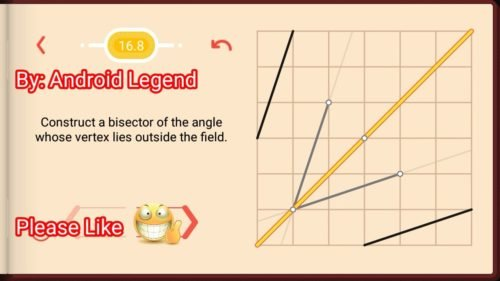• Pythagorea Level 16.9: Construct a bisector of the angle Whose vertex lies outside the field.• Pythagorea Level 16.10: Construct an angle bisector of the given angle.• Pythagorea Level 16.11: Construct the incenter of the triangle.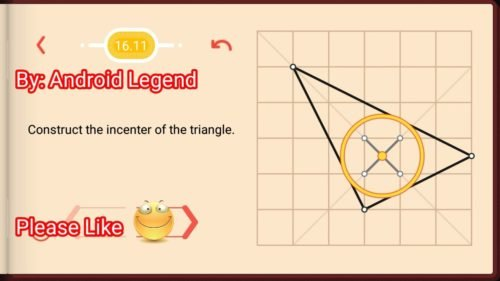• Pythagorea Level 16.12: Construct an angle bisector of the given angle.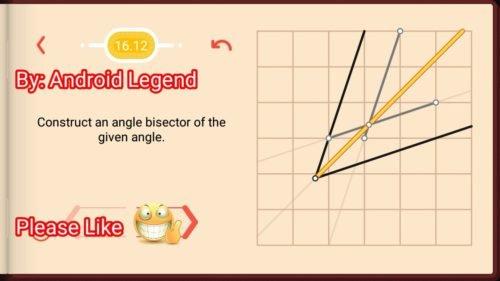• Pythagorea Level 16.13: Construct a bisector of the angle Whose vertex lies outside the field.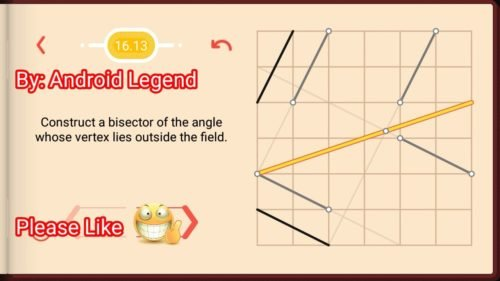• Pythagorea Level 16.14: Construct the incenter of the triangle.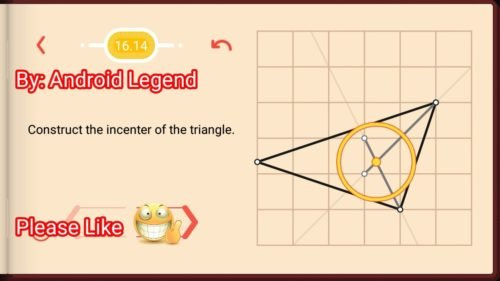• Pythagorea Level 16.15: Construct an angle bisector of the given angle.• Pythagorea Level 16.16: Construct an angle bisector of the given angle.Feel free to comment below if you have any doubts regarding the solutions. I will try to help you guys. All other levels are posted on this blog please visit them too.

# Pythagorea Level 15.1 15.2 15.3 15.4 15.5 15.6 15.7 15.8 15.9 15.10 15.11 15.12 15.13 15.14 Solution/AnswersPythagorea is android/iOS app developed by Horis International Limited. Solutions hints and answers to pythagorea are available in this post scroll down to find solutions to all the levels.

This game is mostly focused on geometric puzzles and construction. the workspace is divided into grids to draw lines. You should know all the basic Math operations. All lines and shapes are drawn on a grid whose cells are squares. Most of the game levels can be answered using natural intuition and bye some basic laws of geometry.

You have to connect points on the grid using straight lines to construct an element, you can even use intersection points to draw. some levels are very easy some are of medium difficulty and some are very hard to solve, that’s why I am providing solutions to all the problems.

If you have forgotten basic course you did in your elementary education, this game is for you to revise all the concept using a single game or if you don’t know any of the geometry this game features “i” button from where you can learn about all the shapes and geometry and then play this game to enhance your geometry skill.And do not miss your chance to familiarize children with mathematics. Pythagorea is an excellent way to make friends with geometry and benefit from spending time together.

If you are here for levels other than ‘Distance’ Go to directory of all other levels at : http://www.puzzlegamemaster.com/pythagorea-all-answers-solutions/

• Pythagorea Level 15.1: Construct all lines through the point C that lie at equal distance from the points A and B.• Pythagorea Level 15.2: Construct all lines through the point C that lie at equal distance from the points A and B.• Pythagorea Level 15.3: Mark all nodes that lie two times farther from the point Athan from the point B.• Pythagorea Level 15.4: Construct all lines through the point C that lie at equal distance from the points A and B.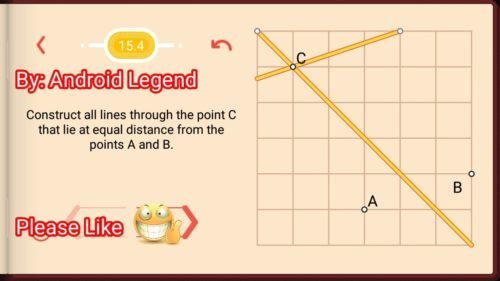• Pythagorea Level 15.5: Construct all lines through the point C that lie at equal distance from the points A and B.• Pythagorea Level 15.6: Mark all nodes that lie two times farther from the point Athan from the point B.• Pythagorea Level 15.7: Mark all nodes that lie two times farther from the point Athan from the point B.• Pythagorea Level 15.8: Construct a point C on the given line such that the sum of lengths AC and CB is minimal.• Pythagorea Level 15.9: Construct a point C on the given line such that the sum of lengths AC and CB is minimal.• Pythagorea Level 15.10: Construct a point C on the given line such that the sum of lengths AC and CB is minimal.• Pythagorea Level 15.11: Construct a point on the line that lies at equal distance from the given two points.• Pythagorea Level 15.12: Construct a point on the line that lies at equal distance from the given two points.• Pythagorea Level 15.13: Construct a point on the line that lies at equal distance from the given two points.• Pythagorea Level 15.14: Construct a point on the line that lies at equal distance from the given two points.Feel free to comment below if you have any doubts regarding the solutions. I will try to help you guys. All other levels are posted on this blog please visit them too.

# Pythagorea Level 14.1 14.2 14.3 14.4 14.5 14.6 14.7 14.8 14.9 Solution/AnswersPythagorea is android/iOS app developed by Horis International Limited. Solutions hints and answers to pythagorea are available in this post scroll down to find solutions to all the levels.

This game is mostly focused on geometric puzzles and construction. the workspace is divided into grids to draw lines. You should know all the basic Math operations. All lines and shapes are drawn on a grid whose cells are squares. Most of the game levels can be answered using natural intuition and bye some basic laws of geometry.

You have to connect points on the grid using straight lines to construct an element, you can even use intersection points to draw. some levels are very easy some are of medium difficulty and some are very hard to solve, that’s why I am providing solutions to all the problems.

If you have forgotten basic course you did in your elementary education, this game is for you to revise all the concept using a single game or if you don’t know any of the geometry this game features “i” button from where you can learn about all the shapes and geometry and then play this game to enhance your geometry skill.And do not miss your chance to familiarize children with mathematics. Pythagorea is an excellent way to make friends with geometry and benefit from spending time together.

If you are here for levels other than ‘Length and Proportions’ Go to directory of all other levels at : http://www.puzzlegamemaster.com/pythagorea-all-answers-solutions/

• Pythagorea Level 14.1: Construct a point on the segment that divides it in the ratio 2:3.• Pythagorea Level 14.2: Construct a point that divides the polyline into two parts of equal length.• Pythagorea Level 14.3: Construct a point that divides the polyline into two parts of equal length.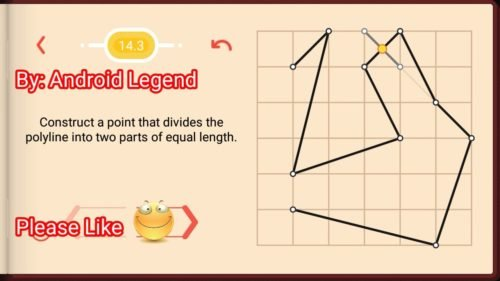• Pythagorea Level 14.4: Construct a point that divides the polyline into two parts of equal length.• Pythagorea Level 14.5: Construct a point on the segment that divides it in the ratio 2:3.• Pythagorea Level 14.6: Construct two points that divide the segment into three equal parts.• Pythagorea Level 14.7: Construct a point on the given ray at distance 1 from the point A.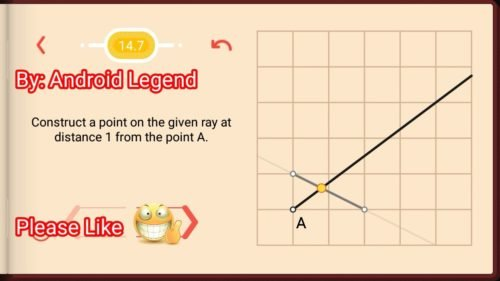• Pythagorea Level 14.8: Construct the midpoint of the segment
AB.• Pythagorea Level 14.9: Construct the midpoint of the segment
AB.Feel free to comment below if you have any doubts regarding the solutions. I will try to help you guys. All other levels are posted on this blog please visit them too.

# Pythagorea Level 13.1 13.2 13.3 13.4 13.5 13.6 13.7 13.8 13.9 13.10 13.11 13.12 13.13 13.14 13.15 Solution/AnswersPythagorea Pythagorean Theorem all levels solved here. Pythagorea is android/iOS app developed by Horis International Limited. Solutions hints and answers to pythagorea are available in this post scroll down to find solutions to all the levels. This game is mostly focused on geometric puzzles and construction. The work space is divided into grids to draw lines. You should know all the basic Math operations. All lines and shapes are drawn on a grid whose cells are squares. Most of the game levels can be answered using natural intuition and by some basic laws of geometry.

You have to connect points on the grid using straight lines to construct an element, you can even use intersection points to draw. Some levels are very easy some are of medium difficulty and some are very hard to solve, that’s why I am providing solutions to all the problems.

If you have forgotten basic course you did in your elementary education, this game is for you to revise all the concept using a single game or if you don’t know any of the geometry this game features “i” button from where you can learn about all the shapes and geometry and then play this game to enhance your geometry skill and do not miss your chance to familiarize children with mathematics. Pythagorea is an excellent way to make friends with geometry and benefit from spending time together.

If you are here for levels other than ‘Pythagorean Theorem’ Go to directory of all other levels at :

##### Pythagorea Pythagorean Theorem all Levels (click on required Level for solution):

Thanks for visiting, If you have any doubts regarding pythagorea, You can comment below.

# Pythagorea Level 12.1 12.2 12.3 12.4 12.5 12.6 12.7 12.8 12.9 12.10 12.11 12.12 12.13 Solution/AnswersPythagorea is android/iOS app developed by Horis International Limited. Solutions hints and answers to pythagorea are available in this post scroll down to find solutions to all the levels.

This game is mostly focused on geometric puzzles and construction. the workspace is divided into grids to draw lines. You should know all the basic Math operations. All lines and shapes are drawn on a grid whose cells are squares. Most of the game levels can be answered using natural intuition and bye some basic laws of geometry.

You have to connect points on the grid using straight lines to construct an element, you can even use intersection points to draw. some levels are very easy some are of medium difficulty and some are very hard to solve, that’s why I am providing solutions to all the problems.

If you have forgotten basic course you did in your elementary education, this game is for you to revise all the concept using a single game or if you don’t know any of the geometry this game features “i” button from where you can learn about all the shapes and geometry and then play this game to enhance your geometry skill.And do not miss your chance to familiarize children with mathematics. Pythagorea is an excellent way to make friends with geometry and benefit from spending time together.

If you are here for levels other than ‘Point Symmetry’ Go to directory of all other levels at : http://www.puzzlegamemaster.com/pythagorea-all-answers-solutions/

Pythagorea Level 12.1: Reflect the point across the point O.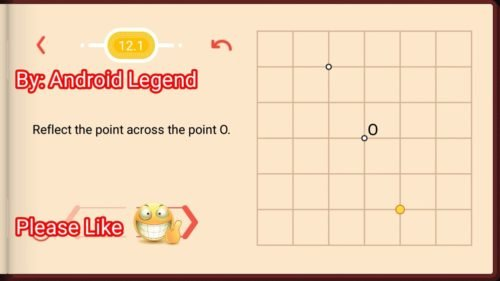Pythagorea Level 12.2: Reflect the segment across the point ΟPythagorea Level 12.3: Construct the center of symmetry of the given figure.Pythagorea Level 12.4: Reflect the polyline across the point O.Pythagorea Level 12.5: Reflect the polyline across the point O.Pythagorea Level 12.6: Reflect the triangle across the point O.Pythagorea Level 12.7: Reflect the triangle across the point O.Pythagorea Level 12.8: Select two points that are symmetric with respect to the point O.Pythagorea Level 12.9: Reflect the figure across the point O.Pythagorea Level 12.10: Reflect the polyline across the point O.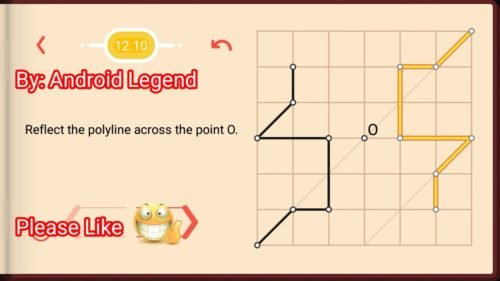Pythagorea Level 12.11: Construct the Center of reflection of the given figures.Pythagorea Level 12.12: Construct the Center of reflection of the given figures.Pythagorea Level 12.13: Construct the Center of reflection of the given figures.Feel free to comment below if you have any doubts regarding the solutions. I will try to help you guys. All other levels are posted on this blog please visit them too.

# Pythagorea Level 11.1 11.2 11.3 11.4 11.5 11.6 11.7 11.8 11.9 11.10 11.11 11.12 Solution/AnswersPythagorea is android/iOS app developed by Horis International Limited. Solutions hints and answers to pythagorea are available in this post scroll down to find solutions to all the levels.

This game is mostly focused on geometric puzzles and construction. the workspace is divided into grids to draw lines. You should know all the basic Math operations. All lines and shapes are drawn on a grid whose cells are squares. Most of the game levels can be answered using natural intuition and bye some basic laws of geometry.

You have to connect points on the grid using straight lines to construct an element, you can even use intersection points to draw. some levels are very easy some are of medium difficulty and some are very hard to solve, that’s why I am providing solutions to all the problems.

If you have forgotten basic course you did in your elementary education, this game is for you to revise all the concept using a single game or if you don’t know any of the geometry this game features “i” button from where you can learn about all the shapes and geometry and then play this game to enhance your geometry skill.And do not miss your chance to familiarize children with mathematics. Pythagorea is an excellent way to make friends with geometry and benefit from spending time together.

If you are here for levels other than ‘Rectangles’ Go to directory of all other levels at : http://www.puzzlegamemaster.com/pythagorea-all-answers-solutions/

Pythagorea Level 11.1: Connect the four given points to get a rectangle.Pythagorea Level 11.2: Construct a rectangle given three of its Vertices.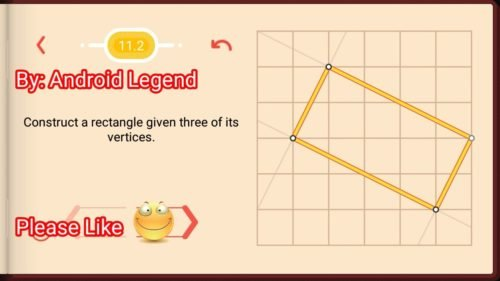Pythagorea Level 11.3: Construct a rectangle given the midpoints of its sides.Pythagorea Level 11.4: Construct a rectangle given the midpoints of its three sides.Pythagorea Level 11.5: Construct a rectangle given a side and the center in the point D.Pythagorea Level 11.6: Construct a rectangle given a side and the center in the point D.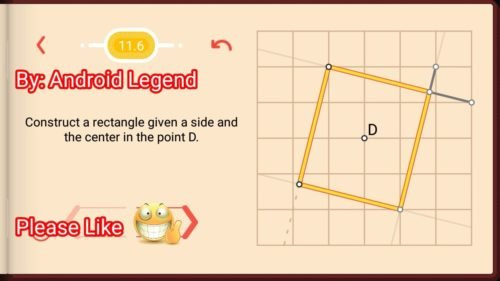Pythagorea Level 11.7: Construct a rectangle given the diagonal and midpoint of the side in the point S.Pythagorea Level 11.8: Construct a rectangle given the diagonal and midpoint of the side in the point S.Pythagorea Level 11.9: Construct a rectangle whose vertices are four of the given points.Pythagorea Level 11.10: Construct a rectangle given the side and midpoint of the opposite side in the point S.Pythagorea Level 11.11: Construct a rectangle given the side and midpoint of the opposite side in the point S.Pythagorea Level 11.12: Construct all rectangles whose vertices are nodes (one of them is in the point A) and the midpoint of the other side in the point S.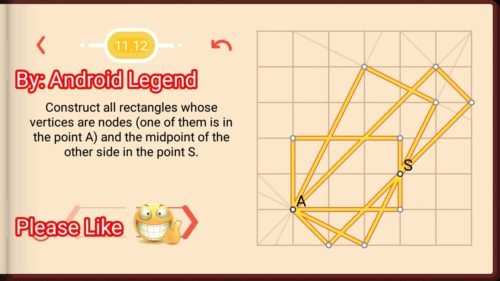Feel free to comment below if you have any doubts regarding the solutions. I will try to help you guys. All other levels are posted on this blog please visit them too.

# Pythagorea Level 10.1 10.2 10.3 10.4 10.5 10.6 10.7 10.8 Solution/AnswersPythagorea is android/iOS app developed by Horis International Limited. Solutions hints and answers to pythagorea are available in this post scroll down to find solutions to all the levels.

This game is mostly focused on geometric puzzles and construction. the workspace is divided into grids to draw lines. You should know all the basic Math operations. All lines and shapes are drawn on a grid whose cells are squares. Most of the game levels can be answered using natural intuition and bye some basic laws of geometry.

You have to connect points on the grid using straight lines to construct an element, you can even use intersection points to draw. some levels are very easy some are of medium difficulty and some are very hard to solve, that’s why I am providing solutions to all the problems.

If you have forgotten basic course you did in your elementary education, this game is for you to revise all the concept using a single game or if you don’t know any of the geometry this game features “i” button from where you can learn about all the shapes and geometry and then play this game to enhance your geometry skill.And do not miss your chance to familiarize children with mathematics. Pythagorea is an excellent way to make friends with geometry and benefit from spending time together.

If you are here for levels other than ‘Circles’ Go to directory of all other levels at : http://www.puzzlegamemaster.com/pythagorea-all-answers-solutions/

Pythagorea Level 10.1: Construct the center of the circle.Pythagorea Level 10.2: Construct a diameter of the circle through the point A.Pythagorea Level 10.3: Construct the center of the circle.Pythagorea Level 10.4: Construct a square whose vertices are nodes and that is circumscribed about the circle.Pythagorea Level 10.5: Construct a square whose vertices are nodes and that is circumscribed about the circle.Pythagorea Level 10.6: Inscribe a square in the circle using the point A as its vertex.Pythagorea Level 10.7: Inscribe a square in the circle using the point A as its vertex.Pythagorea Level 10.8: Construct a square whose vertices are nodes and that is circumscribed about the circle.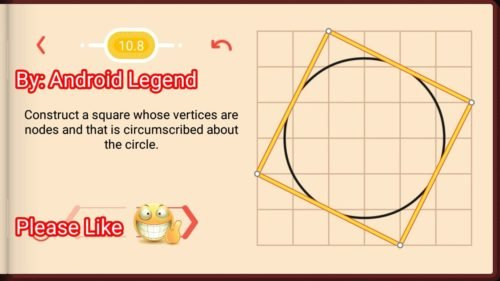Feel free to comment below if you have any doubts regarding the solutions. I will try to help you guys. All other levels are posted on this blog please visit them too.

# Pythagorea Level 9.1 9.2 9.3 9.4 9.5 9.6 9.7 9.8 9.9 9.10 9.11 9.12 9.13 Solution/AnswersPythagorea is android/iOS app developed by Horis International Limited. Solutions hints and answers to pythagorea are available in this post scroll down to find solutions to all the levels.

This game is mostly focused on geometric puzzles and construction. the workspace is divided into grids to draw lines. You should know all the basic Math operations. All lines and shapes are drawn on a grid whose cells are squares. Most of the game levels can be answered using natural intuition and bye some basic laws of geometry.

You have to connect points on the grid using straight lines to construct an element, you can even use intersection points to draw. some levels are very easy some are of medium difficulty and some are very hard to solve, that’s why I am providing solutions to all the problems.

If you have forgotten basic course you did in your elementary education, this game is for you to revise all the concept using a single game or if you don’t know any of the geometry this game features “i” button from where you can learn about all the shapes and geometry and then play this game to enhance your geometry skill.And do not miss your chance to familiarize children with mathematics. Pythagorea is an excellent way to make friends with geometry and benefit from spending time together.

If you are here for levels other than ‘Squares’ Go to directory of all other levels at : http://www.puzzlegamemaster.com/pythagorea-all-answers-solutions/

Pythagorea Level 9.1: Connect the four given points to get a Square.Pythagorea Level 9.2: Construct a Square using the given segment as a side.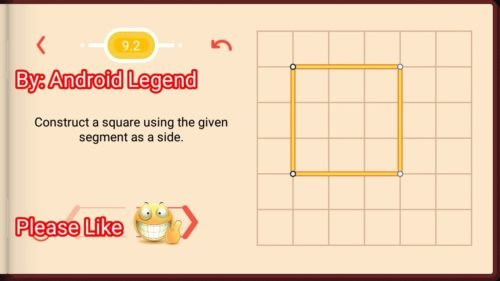Pythagorea Level 9.3: Construct a Square using the given segment as a side.Pythagorea Level 9.4: Construct a square whose two opposite vertices are given.Pythagorea Level 9.5: Construct a square whose two opposite vertices are given.Pythagorea Level 9.6: Construct a square given the midpoints of its sides.Pythagorea Level 9.7: Construct a square given the midpoints of its sides.Pythagorea Level 9.8: Construct a square given the midpoints of its sides.Pythagorea Level 9.9: Inscribe a square in the given one using the point A as its vertex.Pythagorea Level 9.10: Inscribe a square in the given one using the point A as its vertex.Pythagorea Level 9.11: Construct a square whose two opposite vertices are given.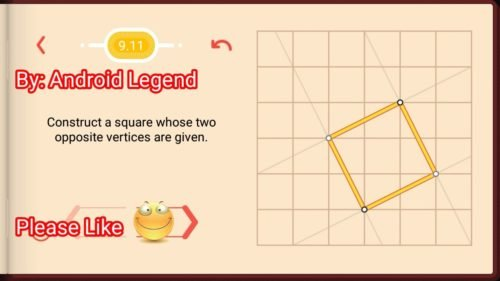Pythagorea Level 9.12: Construct a square whose two opposite vertices are given.Pythagorea Level 9.13: Construct all squares with two of the vertices given.Feel free to comment below if you have any doubts regarding the solutions. I will try to help you guys. All other levels are posted on this blog please visit them too.

# Pythagorea Level 8.1 8.2 8.3 8.4 8.5 8.6 8.7 8.8 8.9 8.10 Solution/AnswersPythagorea Trapezoids all levels solved here. Pythagorea is android/iOS app developed by Horis International Limited. Solutions hints and answers to pythagorea are available in this post scroll down to find solutions to all the levels. This game is mostly focused on geometric puzzles and construction. The work space is divided into grids to draw lines. You should know all the basic Math operations. All lines and shapes are drawn on a grid whose cells are squares. Most of the game levels can be answered using natural intuition and by some basic laws of geometry.

You have to connect points on the grid using straight lines to construct an element, you can even use intersection points to draw. Some levels are very easy some are of medium difficulty and some are very hard to solve, that’s why I am providing solutions to all the problems.

If you have forgotten basic course you did in your elementary education, this game is for you to revise all the concept using a single game or if you don’t know any of the geometry this game features “i” button from where you can learn about all the shapes and geometry and then play this game to enhance your geometry skill and do not miss your chance to familiarize children with mathematics. Pythagorea is an excellent way to make friends with geometry and benefit from spending time together.

If you are here for levels other than ‘Trapezoids’ Go to directory of all other levels at :#### Pythagorea Trapezoids all Levels (click on required Level for solution):

Thanks for visiting, If you have any doubts regarding Pythagorea, You can comment below.

# Pythagorea Level 7.1 7.2 7.3 7.4 7.5 7.6 7.7 7.8 7.9 7.10 Solution/AnswersPythagorea Parallelogram all levels solved here. Pythagorea is android/iOS app developed by Horis International Limited. Solutions hints and answers to pythagorea are available in this post scroll down to find solutions to all the levels. This game is mostly focused on geometric puzzles and construction. The work space is divided into grids to draw lines. You should know all the basic Math operations. All lines and shapes are drawn on a grid whose cells are squares. Most of the game levels can be answered using natural intuition and by some basic laws of geometry.

You have to connect points on the grid using straight lines to construct an element, you can even use intersection points to draw. Some levels are very easy some are of medium difficulty and some are very hard to solve, that’s why I am providing solutions to all the problems.

If you have forgotten basic course you did in your elementary education, this game is for you to revise all the concept using a single game or if you don’t know any of the geometry this game features “i” button from where you can learn about all the shapes and geometry and then play this game to enhance your geometry skill and do not miss your chance to familiarize children with mathematics. Pythagorea is an excellent way to make friends with geometry and benefit from spending time together.Pythagorea Parallelogram all Levels (click on required Level for solution):